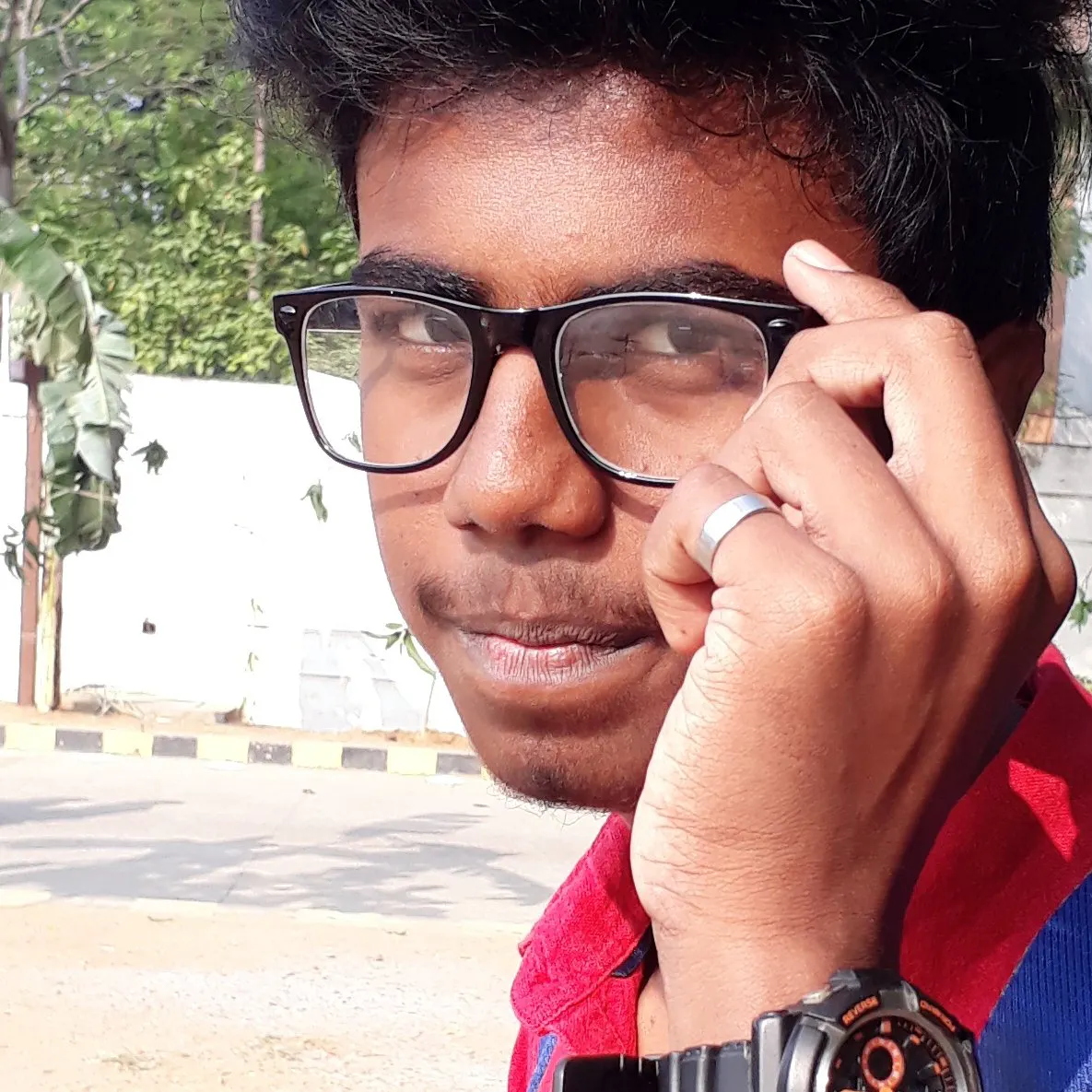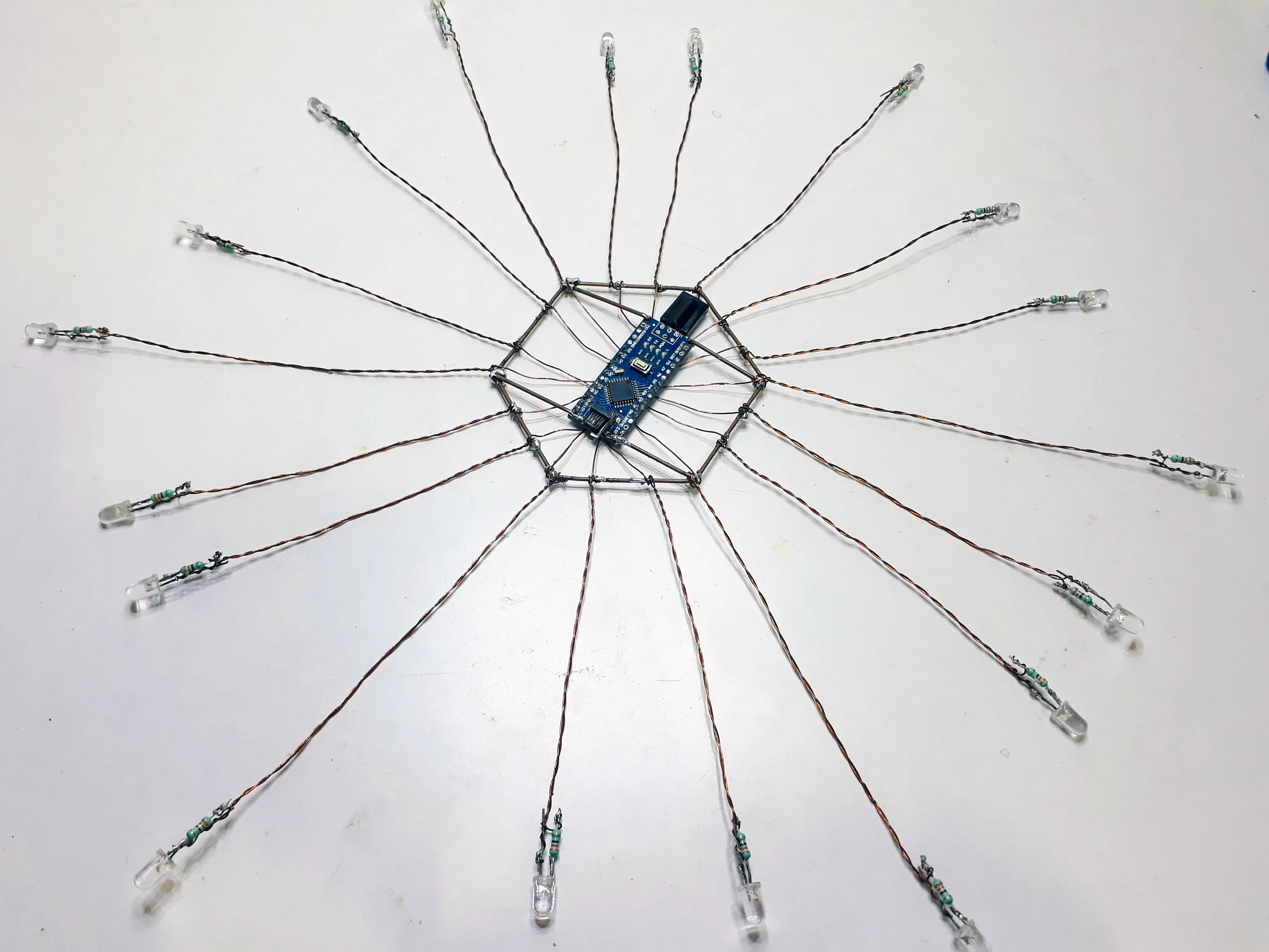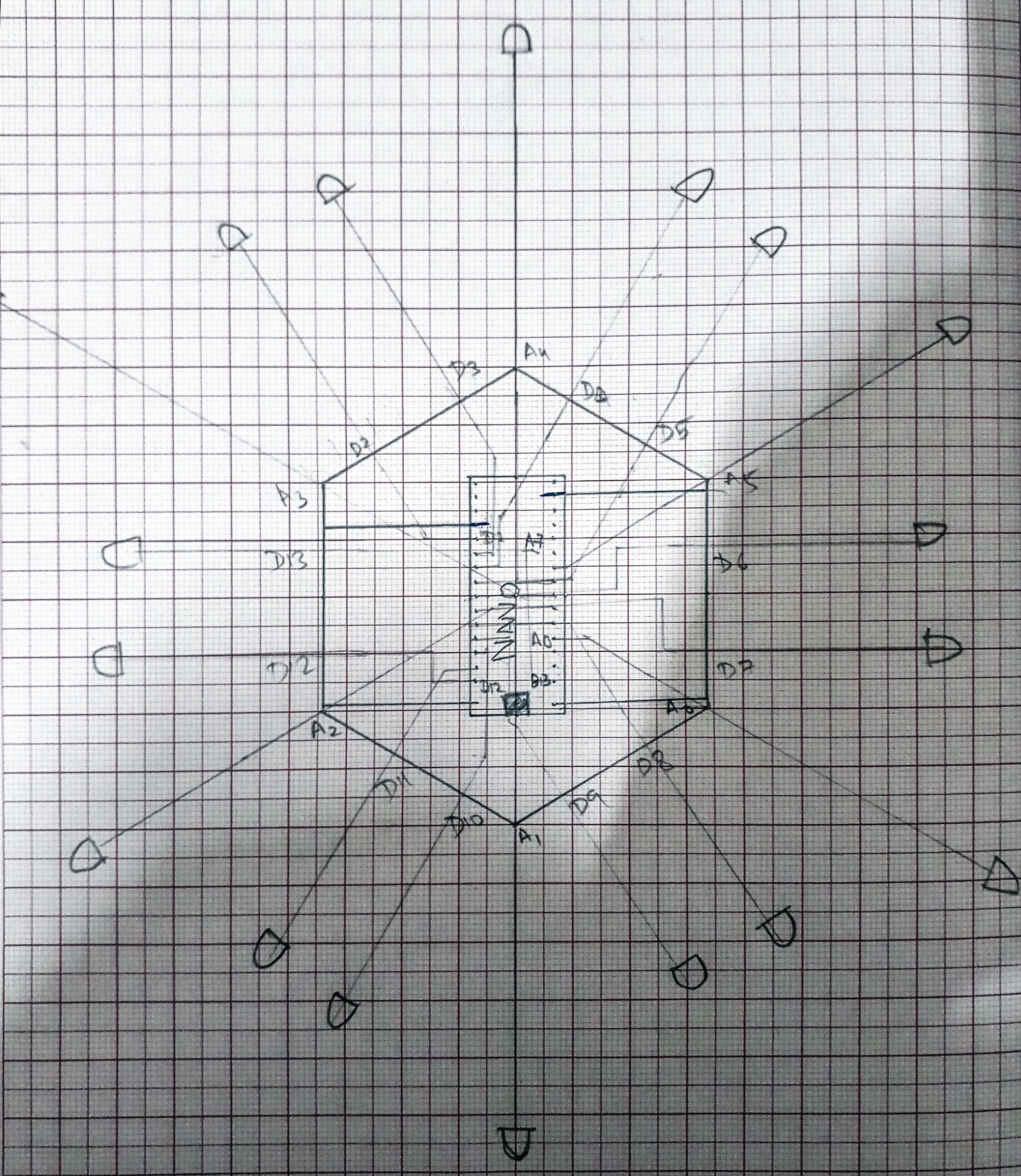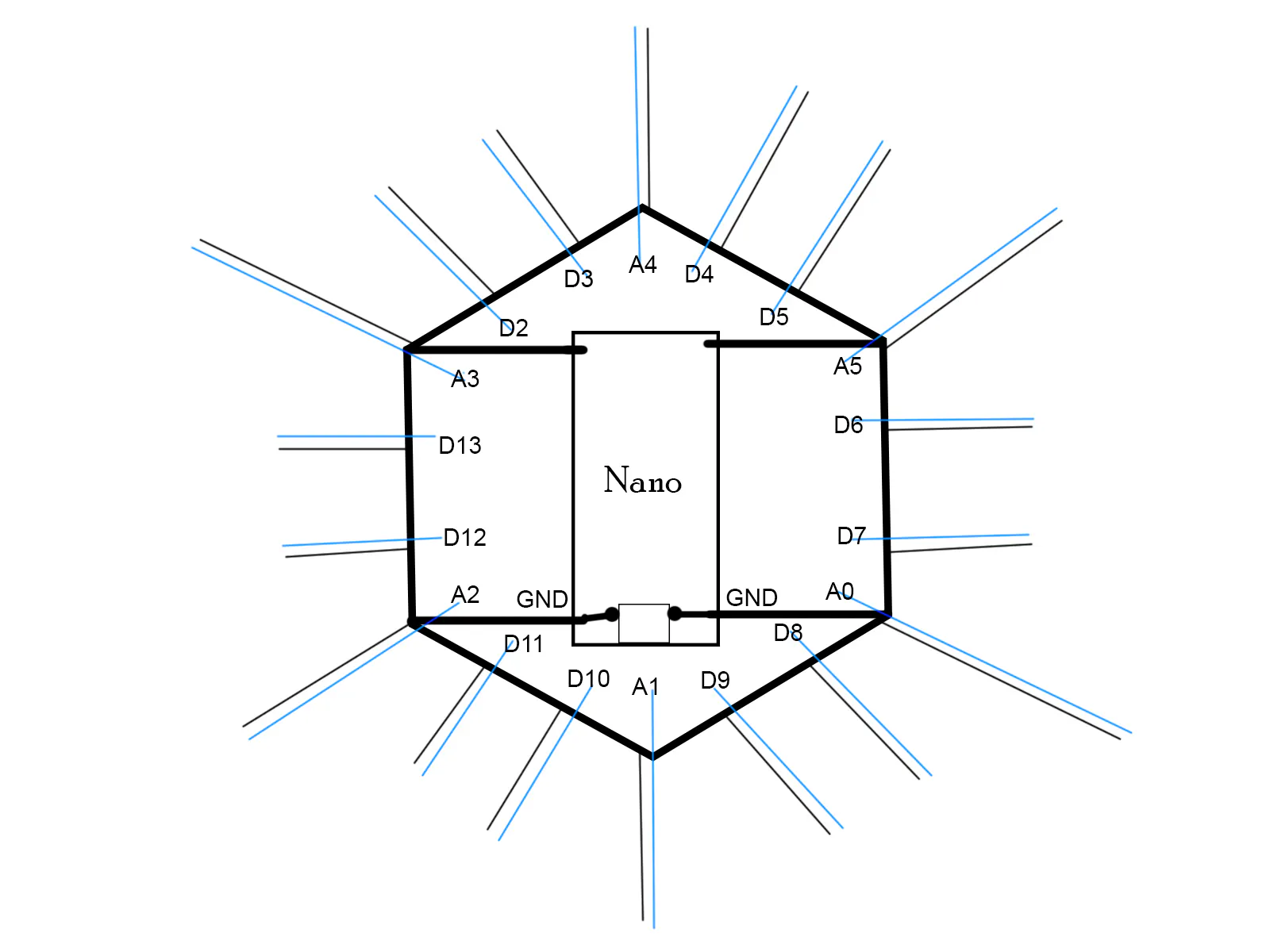# Freeformable Circuit | Real Freeform Circuit!

A freeformable IR remote-controlled LED circuit. An all-in-one applicable DIY light chaser with Arduino-controlled patterns.

IntermediateFull instructions provided6 hours3,132## Things used in this project

### Hardware componentsArduino Nano R3
×1LED (generic)
×1
×1

### Software apps and online servicesArduino IDE

### Hand tools and fabrication machinesSoldering iron (generic)

## Schematics

### Main circuit assemly

You can change pins to your wish. Actually I have circuit design for Particle photon also.### Frame wire connection

the circuit for the code i wrote.## Code

### Freeformable Circuit Code by Devanagaraj

Arduino
Just change the button hex number to your remote numbers.
Open serial moniter in arduino ide to get your hex number of respective remote.
```#include <IRremote.h>
// follow me on insta www.instagram.com/itz_me_stark

int RECV_PIN = 0; //Rx pin
int del= 100;
int led[] = {A4, 4, 5, A5, 6, 7, A0, 8 ,9 , A1 , 10 ,11 ,A2 , 12 ,13 ,A3 ,2 ,3 };

#define val1 1031 // button 7
#define val2 1026 // button 2
#define val3 1027 // button 3
#define val4 1028 // button 4
#define val5 1029 // button 5
#define val6 1223 //power button for full loop effects
#define val7 1030 // button 6
#define val8 1025 // button 1

IRrecv irrecv(RECV_PIN);
decode_results results;

void setup()
{
Serial.begin(9600);
irrecv.enableIRIn();
Serial.println("IR Remote Controlled Freeformable circuit by Devanagaraj");
int i;
for (i=0 ; i<18; i++)
{
pinMode(i, OUTPUT);
digitalWrite(i, LOW);
}
}

void loop()
{
if(irrecv.decode(&results))
{
unsigned int value = results.value;
switch (value)
{
case val1:
if (results.value == val1)
{
for (int i=0 ;i<=2 ;i++)
{
effect1();
}
}
delay(del);

case val2:
if (results.value == val2)
{
for (int i=0 ;i<=2 ;i++)
{
effect2();
}
}
delay(del);

case val3:
if (results.value == val3)
{
for (int i=0;i<=4;i++)
{
effect3();
}
irrecv.resume();
}
delay(del);

case val4:
if (results.value == val4)
{
for (int i=0;i<=4;i++)
{
effect4();
}
irrecv.resume();
}
delay(del);

case val5:
if (results.value == val5)
{
for (int i=0;i<=4;i++)
{
effect5();
}
irrecv.resume();
}
delay(del);

case val6:
if (results.value == val6)
{
effect1();
effect2();
effect3();
effect4();
effect5();
loop();
irrecv.resume();
}
delay(del);

case val7:
if (results.value == val7)
{
for(int i=0;i<=4;i++)
{
effect6();
effect6();
}
}
delay(del);

case val8:
if (results.value == val8)
{
effect7();
effect7();
effect7();
}
delay(del);
}

Serial.println(value);
irrecv.resume();
}
}

void effect1()
{
int x;
for (x = 0; x < 18; x++)
{
digitalWrite(led[x], HIGH);
delay(del);
}
delay(del);
for (x = 0; x < 18; x++)
{
digitalWrite(led[x], LOW);
delay(del);
}
}

void effect2()
{
int x;
for (x = 0; x < 18; x++)
{
digitalWrite(led[x], HIGH);
delay(del);
digitalWrite(led[x], LOW);
}
delay(del);
for (x = 18; x > 0; x--)
{
digitalWrite(led[x], HIGH);
delay(del);
digitalWrite(led[x], LOW);
}
}

void effect3()
{
int x;
for (x = 0; x < 18; x++)
{
digitalWrite(led[x], HIGH);
digitalWrite(led[x + 1], HIGH);
delay(del);
digitalWrite(led[x], LOW);
digitalWrite(led[x + 1], LOW);
}
delay(del);
for (x = 18; x > 0; x--)
{
digitalWrite(led[x], HIGH);
digitalWrite(led[x - 1], HIGH);
delay(del);
digitalWrite(led[x], LOW);
digitalWrite(led[x - 1], LOW);
}
}

void effect4()
{
int x;
for (x = 0; x <= 8; x++)
{
digitalWrite(led[x], HIGH);
delay(del);
digitalWrite(led[17 - x], HIGH);
delay(del);
}
delay(1000);
for (x = 8; x >= 0; x--)
{
digitalWrite(led[x], LOW);
delay(del);
digitalWrite(led[17 - x], LOW);
}
}

void effect5()
{
int x;
for (x = 8; x >= 0; x--)
{
digitalWrite(led[x], HIGH);
delay(del);
digitalWrite(led[17 - x], HIGH);
delay(del);
}

delay(1000);
for (x = 0; x <= 8; x++)
{
digitalWrite(led[x], LOW);
delay(del);
digitalWrite(led[17 - x], LOW);
}
}

void effect6()
{
int x;
x = random(17);

digitalWrite (led[x] , HIGH);
delay(del);
digitalWrite(led[x] , LOW);
loop();
}

void effect7()
{
digitalWrite (led[0&2],HIGH);
}
```

## Credits

### Devanagaraj

2 projects • 7 followers
Mechanical engineering student. Maker from India. Keen to learn and Change.